# Keisler-Shelah isomorphism theorem

(diff) ← Older revision | Latest revision (diff) | Newer revision → (diff)

Interpretations for a first-order languageare said to be elementarily equivalent (in) provided that they make exactly the same sentences intrue (cf. also Interpretation). The Keisler–Shelah isomorphism theorem provides a characterization of elementary equivalence: interpretations forare elementarily equivalent if and only if they have isomorphic ultrapowers (cf. also Ultrafilter).

This theorem was formulated and proved by H.J. Keisler in 1961 [a2]. Keisler gave a second proof in 1964 using saturated ultrapowers [a3]. Both proofs use the generalized continuum hypothesis (GCH). In 1971 S. Shelah gave a third proof [a5]. This proof avoids the generalized continuum hypothesis.

Given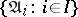, a non-empty family of interpretations for, andan ultrafilter on, the ultraproductof the family is the quotient system on the direct product of the family induced by. When there is a fixed interpretation,, such that each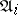coincides with,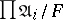is denoted by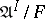and is called an ultrapower of. It follows from results of J. Łos [a4] thatand any of its ultrapowers are elementarily equivalent (the Łos isomorphism theorem). Hence, interpretations with isomorphic ultrapowers are elementarily equivalent.

Letbe an infinite cardinal no smaller than the cardinality of the set of sentences in, and letand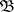be interpretations forof cardinality less than or equal to. Let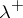denote the cardinal successor of. Keisler showed (assuming that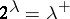) thatandare elementarily equivalent if and only if there are ultrafiltersonsuch that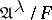and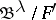are isomorphic.

Letbe as above and letbe the least cardinal such that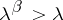. Shelah showed (without assuming that) that there is an ultrafilteronsuch that, givenand, elementarily equivalent interpretations of cardinality less than,and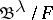are isomorphic.

The motivation for Keisler's results can be found in a programme propounded by A. Tarski [a6]: to provide characterizations of meta-mathematical notions in "purely mathematical terms" . A discussion of this programme and its history can be found in [a7]. To appreciate what was intended here, recall G. Birkhoff's 1935 characterization [a1] of the classes of models of sets of equations (the equational classes): A class of algebras is an equational class if and only if it is closed under subalgebras, homomorphic images and direct products. This result characterizes equational classes without mentioning equations.

Amongst the consequences of the Keisler–Shelah isomorphism theorem is a comparable "mathematical" characterization of the classes of models of sentences in. Given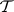, a class of interpretations for,is an elementary class provided that there is a sentence inwhose models are exactly the members of;is an elementary class in the wider sense provided that there is a set of sentences inwhose models are exactly the members of. It follows from the compactness theorem thatis an elementary class if and only if bothand its complement (relative to the class of interpretations for) are elementary classes in the wider sense. Keisler [a2] showed (assuming GCH) that:

1)is an elementary class in the wider sense, provided thatis closed under isomorphic images and ultraproducts and the complement ofis closed under ultrapowers;

2)is an elementary class if and only if bothand its complement are closed under isomorphic images and ultraproducts.

Whilst Keisler's proof of this result used the generalized continuum hypothesis, its use was restricted to establishing that elementarily equivalent interpretations have isomorphic ultrapowers. Hence, by eliminating GCH in the proof of the latter result, Shelah also eliminated the use of GCH from Keisler's characterization of elementary classes.

How to Cite This Entry:
Keisler-Shelah isomorphism theorem. Encyclopedia of Mathematics. URL: http://encyclopediaofmath.org/index.php?title=Keisler-Shelah_isomorphism_theorem&oldid=11976
This article was adapted from an original article by G. Weaver (originator), which appeared in Encyclopedia of Mathematics - ISBN 1402006098. See original article